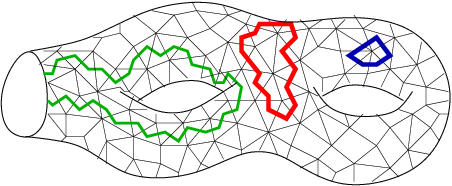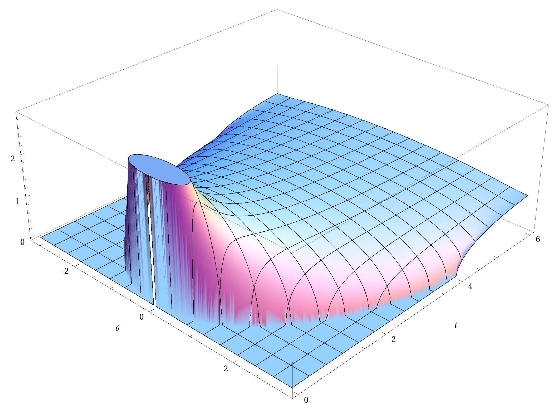# GranMa 2011

## Institut Henri Poincaré, Paris

General

Announcement

Participants

Practical details

Registration

Schedule

Guillaume Aubrun, Universitť Lyon 1:

## Thresholds for random quantum states

We consider a model of random states on $H=\C^d \otimes \C^d$ which are obtained by partial tracing a random Haar-distributed pure state on $H \otimes \C^s$. We study the following questions: are these random states entangled ? do they have PPT (positive partial transpose) ? The answers depend on the relative values of the dimensions $d$ and $s$. We use tools from high-diemsional convex geometry and random matrix theory. In particular we prove the following result about Wishart matrices: let $W$ be a Wishart random matrix of size $d^2 \times d^2$, considered as a block matrix with $d \times d$ blocks. Let Y be the matrix obtained by transposing each block of W. We prove that the empirical eigenvalue distribution of Y approaches a (non-centered) semicircular distribution when d tends to infinity. (based on joint work with S. Szarek and D. Ye)